Like   Tweet   Pin   +1   in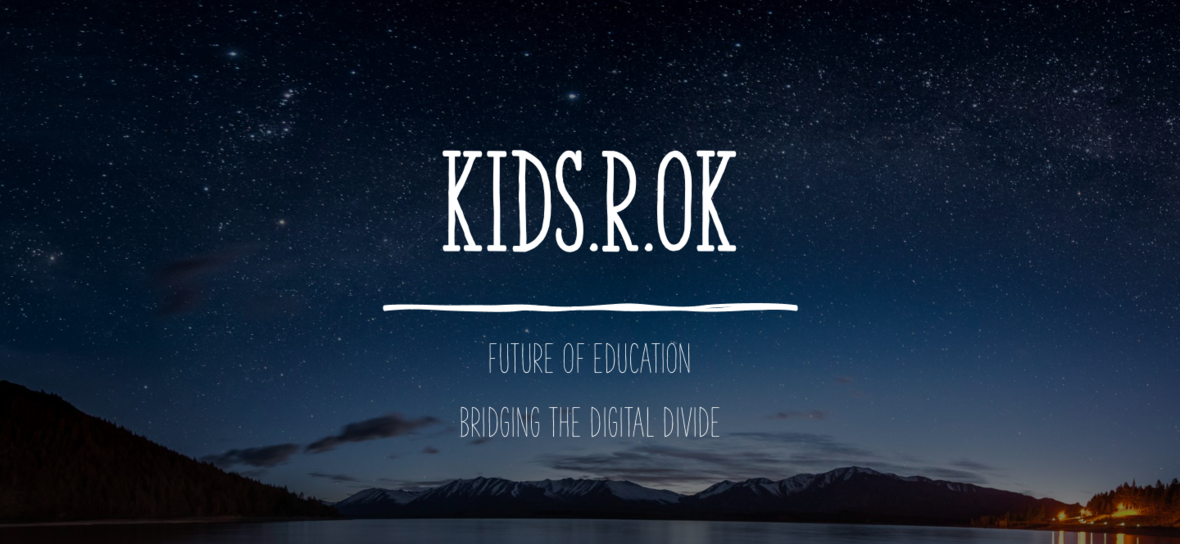table div table+table div table{width:100%;padding:0}table div table+table div table img{width:96.23%;padding:0;float:none}table div table+table div table td{width:100%;padding:0 1.88% 18px}/* styles */## Together We Strive - 2020

 /* styles */ Dear Friend of kids.R.ok Hope you are all well and safe during this unprecedented year. 2020 will probably go down as a pivotal year in history for many things including realizations and reflections. What actions we take now will determine the course of tomorrow and the lessons we can learn from today will create a better future. In that note, we have focused on quickly adapting and working to bridge the digital divide that many children and families face in the communities we serve with online classes and initiatives to maintain continuity of learning for our children. kids.R.ok would like to specially thank our team in India, led by our Project Manager, Evangelin Grace and coordinated by our teachers under the leadership of our Indian Director Sunjeet Sudhir, to keep all our projects on track and ensure the health and safety on the ground despite challenges. The children and their families have faced compounding effects of this pandemic with spending months under lockdown, economic hardships and losses with even one of our children's father unfortunately losing his life due to COVID-19. Despite these challenges our community and children have been resilient and worked to continue their pursuit of education. Thanks to the positive attitude of our team which has indeed trickled down to the children.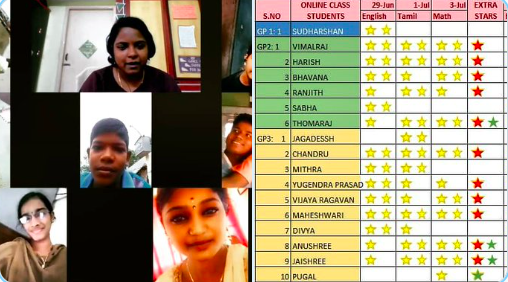We encourage more children to participate in the online class through a star system for completing assignments.

We have rapidly adapted our programs for virtual settings using Zoom and Whatsapp platforms since our onsite centers had to temporary close in early March due to the pandemic. The children have continued to thrive in their learning during this time and we have received many positive feedback from their parents and families about their children learning from home.

## Math & Science with interactive learning:

Exploring and learning about space! We are using interactive educational tools to make science and math easy & fun to learn.

 table div table+table+table+table+table+table+table+table div table td,table.module-7{width:100%;padding:0}table div table+table+table+table+table+table+table+table div table{width:100%;float:none;margin-left:auto;margin-right:auto;padding:0}table div table+table+table+table+table+table+table+table div table a{border:0 none;text-decoration:none}table div table+table+table+table+table+table+table+table div table img{width:100%!important;border:0 none;text-decoration:none}/* styles */
 table.module-8{width:99.81%;padding:0}table div table+table+table+table+table+table+table+table+table div table{width:99.81%;float:none;margin-left:auto;margin-right:auto;padding:0}table div table+table+table+table+table+table+table+table+table div table a{border:0 none;text-decoration:none}table div table+table+table+table+table+table+table+table+table div table img{width:100%!important;border:0 none;text-decoration:none}table div table+table+table+table+table+table+table+table+table div table td{width:100%;padding:0}/* styles */
 /* styles */ Look at some fine artwork by the kids and few videos they sent based on our online extra circular teaching activity ✌

## Stories of Impact

 /* styles */ A positive story we want to share from one of our projects. A young boy named Vignesh was struggling in school who barely passed his 10th grade after many attempts and wanted to dropout. Seeing his peers drop out and get labor jobs and making money seemed like a path he would want to take over school. His mother was distressed about his plans and approached us to intervene. kids.R.ok team took the time to have several counselling meeting with him and his parents for his to see the long term advantages of investing in his education now for a better future for him. We are glad to say that he took our advise and with the continued hard work and extra support from our teachers he has graduated high school this year and was admitted to a University to study further. This is a reflection of our motto of No Child Left Behind!
 table div table+table+table+table+table+table+table+table+table+table+table+table+table div table{width:100%;padding:0}table div table+table+table+table+table+table+table+table+table+table+table+table+table div table img{width:96.23%;padding:0;float:none}table div table+table+table+table+table+table+table+table+table+table+table+table+table div table td{width:100%;padding:0 1.88% 18px}/* styles */## COVID-19 Continued Response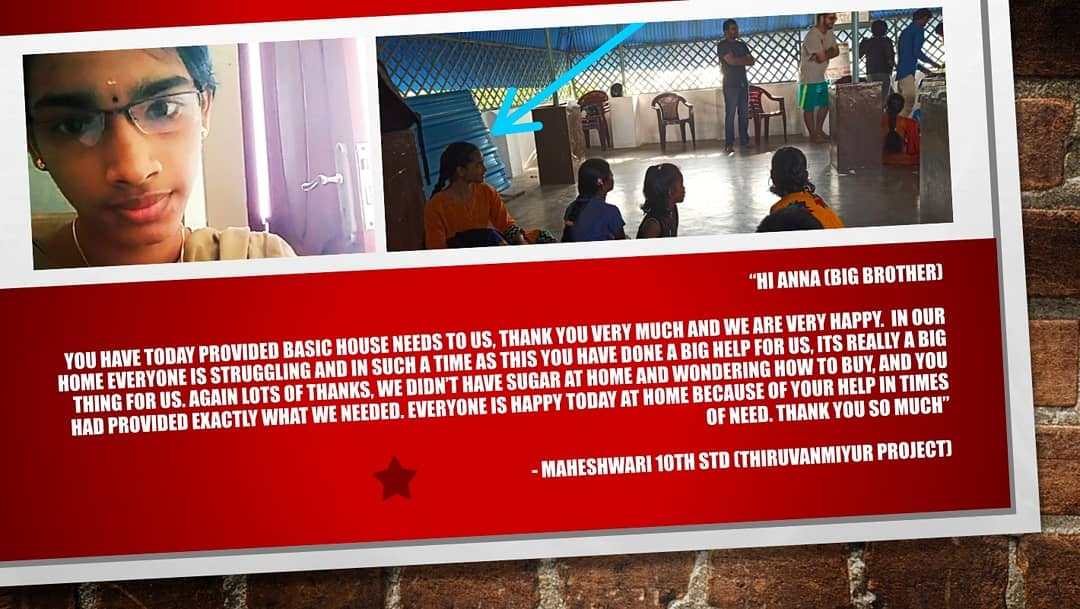"I’m very grateful to all our friends and donors who have really come out in such a generous fashion and provided such support to all our children’s families" Sunjeet Sudhir - Kids.R.ok Co-Founder and Director India

Emergency Food Relief: We would like to thank our well wishers and donors for your continued support this year. We managed to feed 110 daily wage earning families living in the slums where our children come from for two full months.

 table div table+table+table+table+table+table+table+table+table+table+table+table+table+table+table+table div table{width:100%;padding:0}table div table+table+table+table+table+table+table+table+table+table+table+table+table+table+table+table div table img{width:96.23%;padding:0;float:none}table div table+table+table+table+table+table+table+table+table+table+table+table+table+table+table+table div table td{width:100%;padding:0 1.88% 18px}/* styles */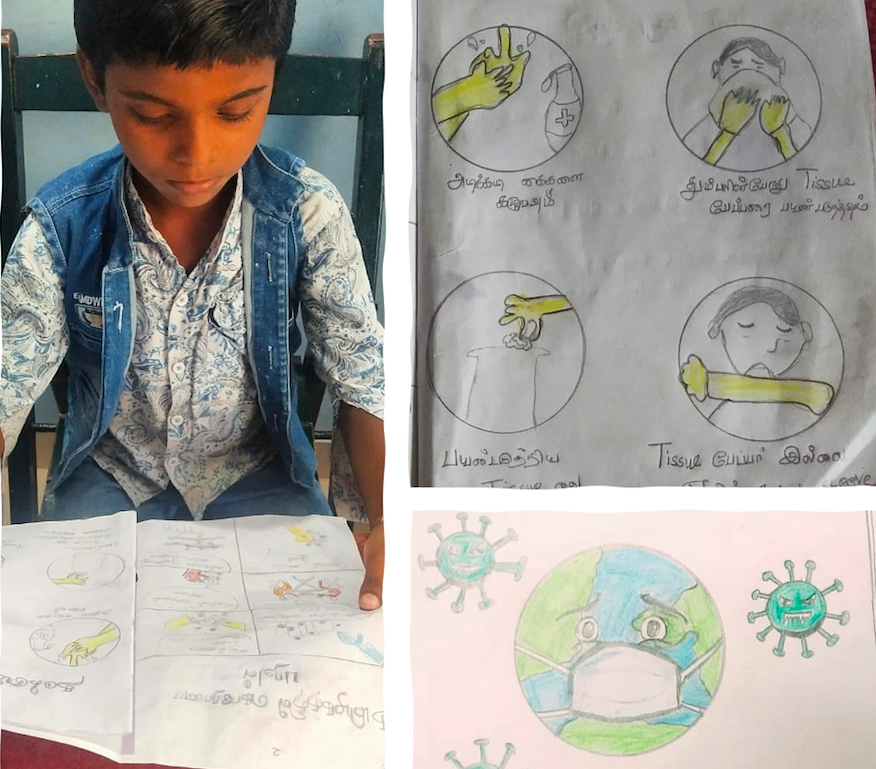Comprehensive Learning Support: We deployed a four phase strategic approach -
* Psycho Social support,
* Online education support,
* Staff development support and
* Economical support.
We ran several online career guidance and counselling sessions for both children and parents. We provided extra resources for our teachers to sustain continued interest and provide an environment for the children to learn despite the current limitations.

 table div table+table+table+table+table+table+table+table+table+table+table+table+table+table+table+table+table+table div table{width:100%;padding:0}table div table+table+table+table+table+table+table+table+table+table+table+table+table+table+table+table+table+table div table img{width:96.23%;padding:0;float:none}table div table+table+table+table+table+table+table+table+table+table+table+table+table+table+table+table+table+table div table td{width:100%;padding:0 1.88% 18px}/* styles */## Change Makers

 table div table+table+table+table+table+table+table+table+table+table+table+table+table+table+table+table+table+table+table+table div table,table.module-19{width:47.36%;float:left;padding:0}table div table+table+table+table+table+table+table+table+table+table+table+table+table+table+table+table+table+table+table+table div table a{border:0 none;text-decoration:none}table div table+table+table+table+table+table+table+table+table+table+table+table+table+table+table+table+table+table+table+table div table img{width:100%!important;border:0 none;text-decoration:none}table div table+table+table+table+table+table+table+table+table+table+table+table+table+table+table+table+table+table+table+table div table td{width:100%;padding:0 20px 20px 0}/* styles */ Change-makers!! Esther is our teacher at the Saidapet center and her dream is to foster values in our children to create great leaders of our future. It is a privilege to have people like her on our kids.R.ok team. Read more about her story here
 table div table+table+table+table+table+table+table+table+table+table+table+table+table+table+table+table+table+table+table+table+table div table{width:100%;padding:0}table div table+table+table+table+table+table+table+table+table+table+table+table+table+table+table+table+table+table+table+table+table div table img{width:96.23%;padding:0;float:none}table div table+table+table+table+table+table+table+table+table+table+table+table+table+table+table+table+table+table+table+table+table div table td{width:100%;padding:0 1.88% 18px}/* styles */## Mentorship Program

 /* styles */ Kids.R.ok kick started our 'Mentorship Program' this year with a cohort of 16 volunteers. After getting to know the mentors we matched with a mentee from our centers. This program is for our children who are between ages 14-18. We are grateful to have passionate, empathetic and inspiring people to lead the selected kids during our 8 weeks mentoring program.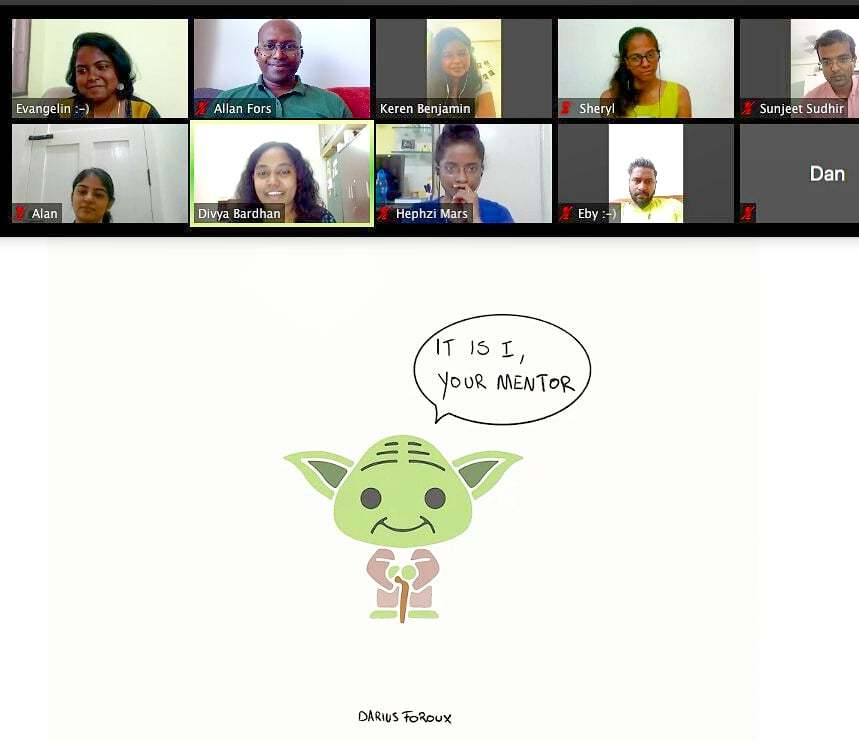table div table+table+table+table+table+table+table+table+table+table+table+table+table+table+table+table+table+table+table+table+table+table+table+table+table div table{width:100%;padding:0}table div table+table+table+table+table+table+table+table+table+table+table+table+table+table+table+table+table+table+table+table+table+table+table+table+table div table img{width:96.23%;padding:0;float:none}table div table+table+table+table+table+table+table+table+table+table+table+table+table+table+table+table+table+table+table+table+table+table+table+table+table div table td{width:100%;padding:0 1.88% 18px}/* styles */## Celebrations & Activities

 /* styles */ Virtual Talent Show
 /* styles */ After a marathon virtual talent show, where the kids in their homes with great enthusiasm showed various skills and talents. We reached out to meet them in person in their homes and give a small gift.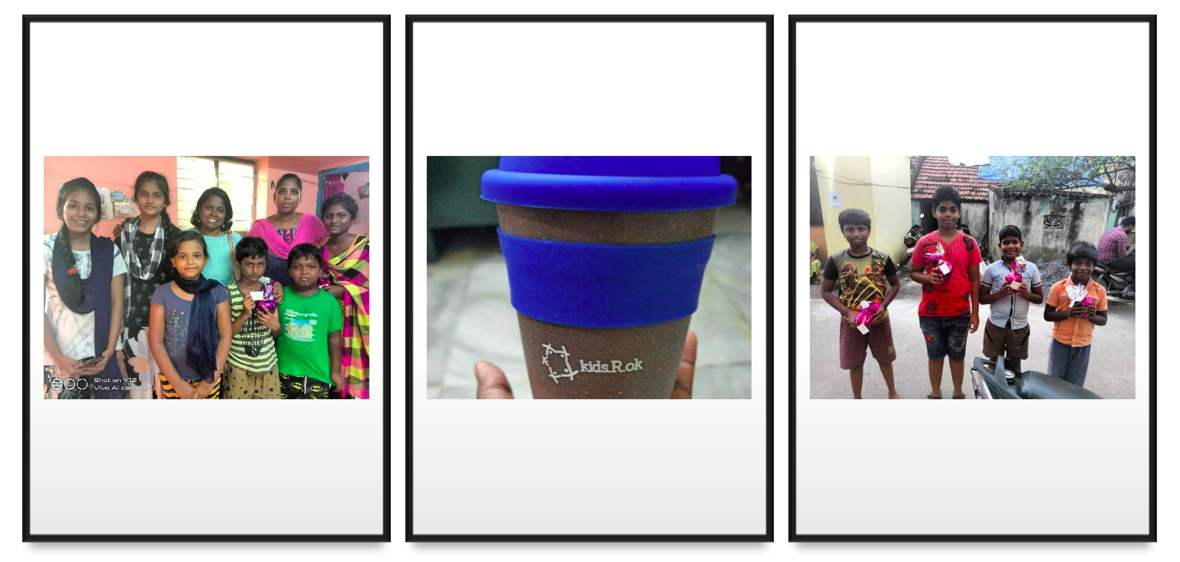A little something to keep the spirits up
 /* styles */ Children's Day - Team Wishes Our Kids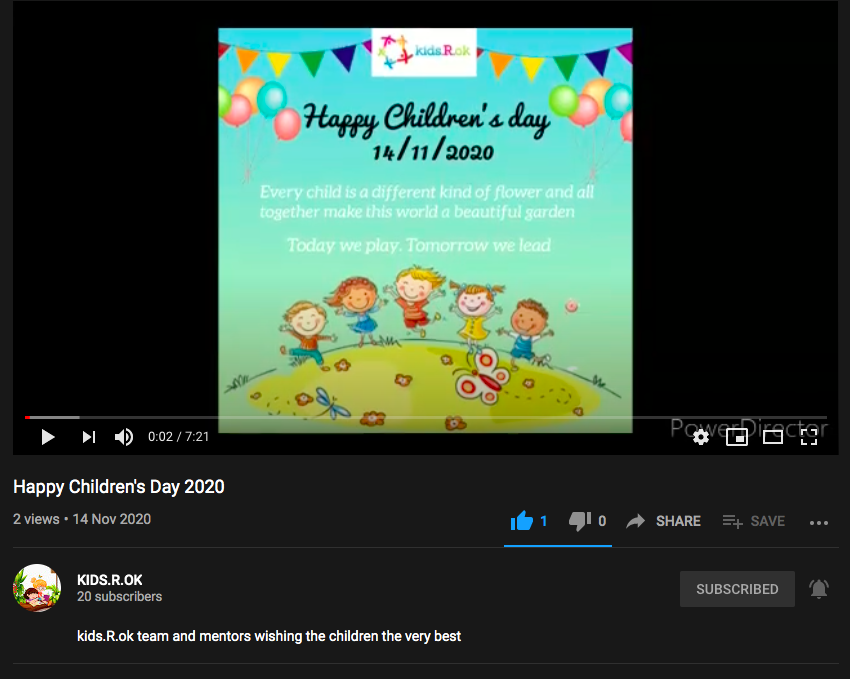YOUTUBE VIDEO - CHECKOUT THE WISHES OF OUR TEAM TO THE CHILDREN
 table div table+table+table+table+table+table+table+table+table+table+table+table+table+table+table+table+table+table+table+table+table+table+table+table+table+table+table+table+table+table+table+table div table{width:100%;padding:0}table div table+table+table+table+table+table+table+table+table+table+table+table+table+table+table+table+table+table+table+table+table+table+table+table+table+table+table+table+table+table+table+table div table img{width:96.23%;padding:0;float:none}table div table+table+table+table+table+table+table+table+table+table+table+table+table+table+table+table+table+table+table+table+table+table+table+table+table+table+table+table+table+table+table+table div table td{width:100%;padding:0 1.88% 18px}/* styles *//* styles */ Teacher Appreciation Day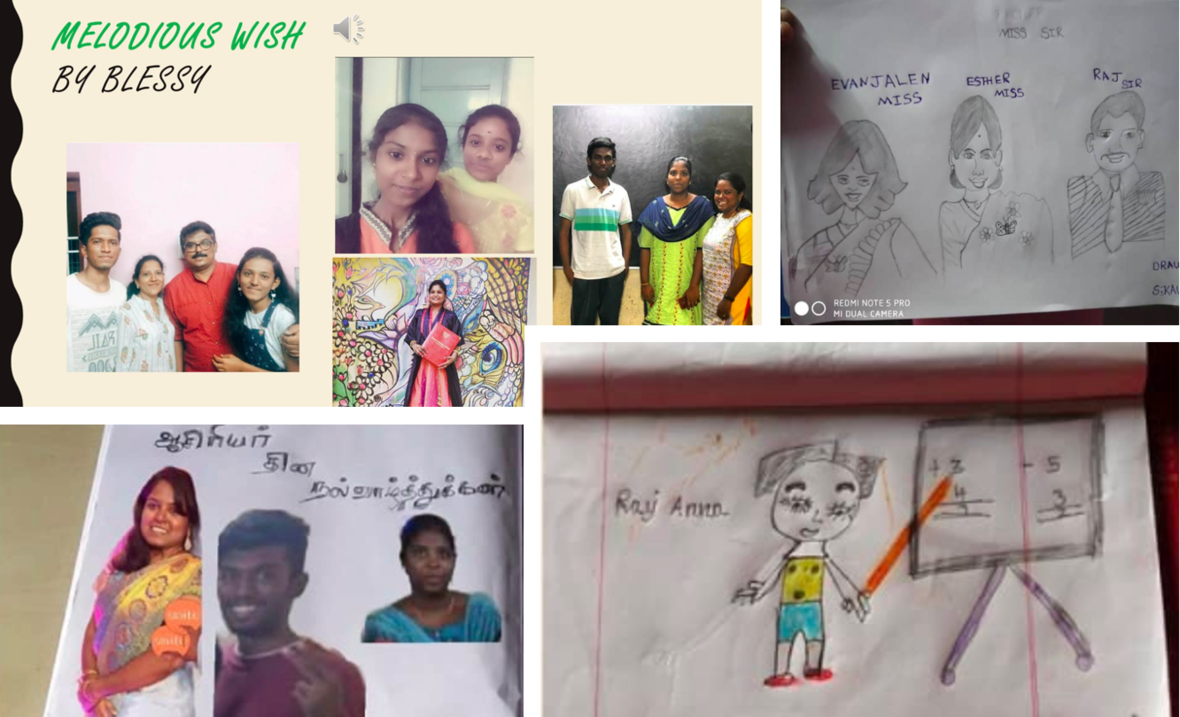Artwork done by our children on Teachers Appreciation Day
 table div table+table+table+table+table+table+table+table+table+table+table+table+table+table+table+table+table+table+table+table+table+table+table+table+table+table+table+table+table+table+table+table+table+table+table div table{width:100%;padding:0}table div table+table+table+table+table+table+table+table+table+table+table+table+table+table+table+table+table+table+table+table+table+table+table+table+table+table+table+table+table+table+table+table+table+table+table div table img{width:96.23%;padding:0;float:none}table div table+table+table+table+table+table+table+table+table+table+table+table+table+table+table+table+table+table+table+table+table+table+table+table+table+table+table+table+table+table+table+table+table+table+table div table td{width:100%;padding:0 1.88% 18px}/* styles */## Thank you

 /* styles */ This year has been a reminder that we can overcome challenges when we work together, remain strong, encouraging each other and showing love and compassion in times of darkness which is very reflective of the how we at kids.R.ok have worked resiliently through these trying times. This wouldn't have been possible without your donations, generosity and immense support! Thank You
 table div table+table+table+table+table+table+table+table+table+table+table+table+table+table+table+table+table+table+table+table+table+table+table+table+table+table+table+table+table+table+table+table+table+table+table+table+table+table+table div table,table.module-38{width:3.77%;float:right;padding:0}table div table+table+table+table+table+table+table+table+table+table+table+table+table+table+table+table+table+table+table+table+table+table+table+table+table+table+table+table+table+table+table+table+table+table+table+table+table+table+table div table a{border:0 none;text-decoration:none}table div table+table+table+table+table+table+table+table+table+table+table+table+table+table+table+table+table+table+table+table+table+table+table+table+table+table+table+table+table+table+table+table+table+table+table+table+table+table+table div table img{width:100%!important;border:0 none;text-decoration:none}table div table+table+table+table+table+table+table+table+table+table+table+table+table+table+table+table+table+table+table+table+table+table+table+table+table+table+table+table+table+table+table+table+table+table+table+table+table+table+table div table td{width:100%;padding:0 0 20px 20px}/* styles */ Consider A Monthly Donation Thank you donors and friends for your contributions to support the children. To consistently support our resilient teachers and educational initiatives for our kids consider donating monthly to kids.R.ok here. With much gratitude, kids.R.ok Team
 table div table+table+table+table+table+table+table+table+table+table+table+table+table+table+table+table+table+table+table+table+table+table+table+table+table+table+table+table+table+table+table+table+table+table+table+table+table+table+table+table div table{width:100%;padding:0}table div table+table+table+table+table+table+table+table+table+table+table+table+table+table+table+table+table+table+table+table+table+table+table+table+table+table+table+table+table+table+table+table+table+table+table+table+table+table+table+table div table img{width:96.23%;padding:0;float:none}table div table+table+table+table+table+table+table+table+table+table+table+table+table+table+table+table+table+table+table+table+table+table+table+table+table+table+table+table+table+table+table+table+table+table+table+table+table+table+table+table div table td{width:100%;padding:0 1.88% 18px}/* styles */